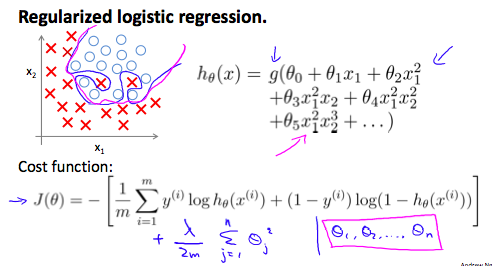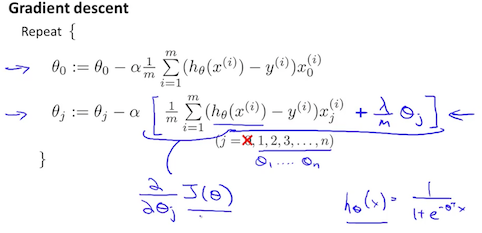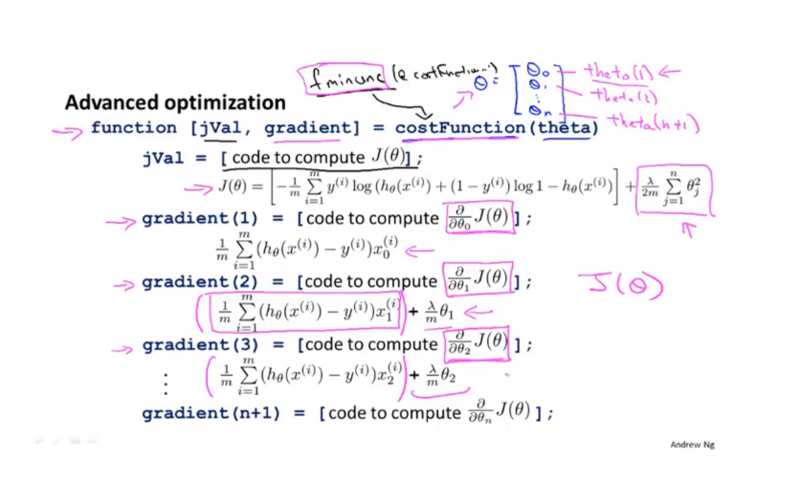## 概念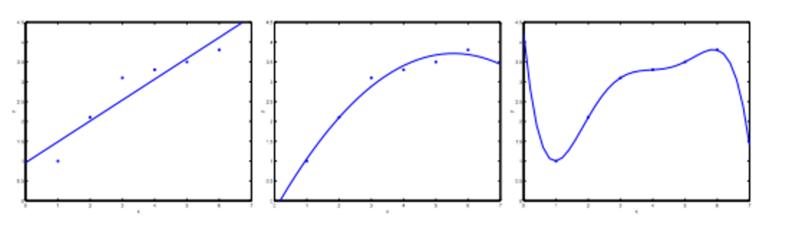1. 减少特征的数量
• 手动确认哪些特征需要保留
• 使用模型选择算法
2. 正则化
• 保留所有的特征，但减小参数 j 的量级
• 正则化在在我们拥有大量的有用的特征时往往能处理的很好

## 代价函数

\frac{1}{2m}\sum_{i=1}^{m}(h_\theta(x^(i)) – y^(i))+1000_\theta_3^2+1000_\theta_4^2

J(\theta) = \frac{1}{2m}[\sum_{i=1}^{m}(h_\theta(x^(i)) – y^(i)) + \lambda\sum_{j=1}^{n}\theta_j^2]

## 线性回归的正则化

\theta_j := \theta_j-\alpha\frac{1}{m}\sum_{i=1}^{m}(h_\theta(x(i)）)-y^(i))x_j^(i)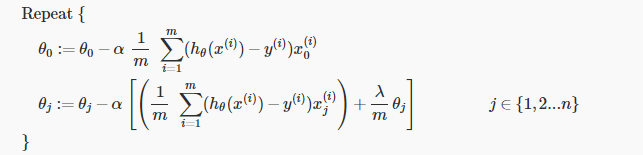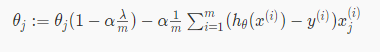\theta = (X^TX)^{-1} X^Ty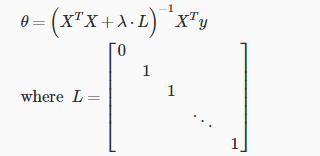## 正则化 logistic 回归